# What the Hex?

October 24, 2022Grant Sander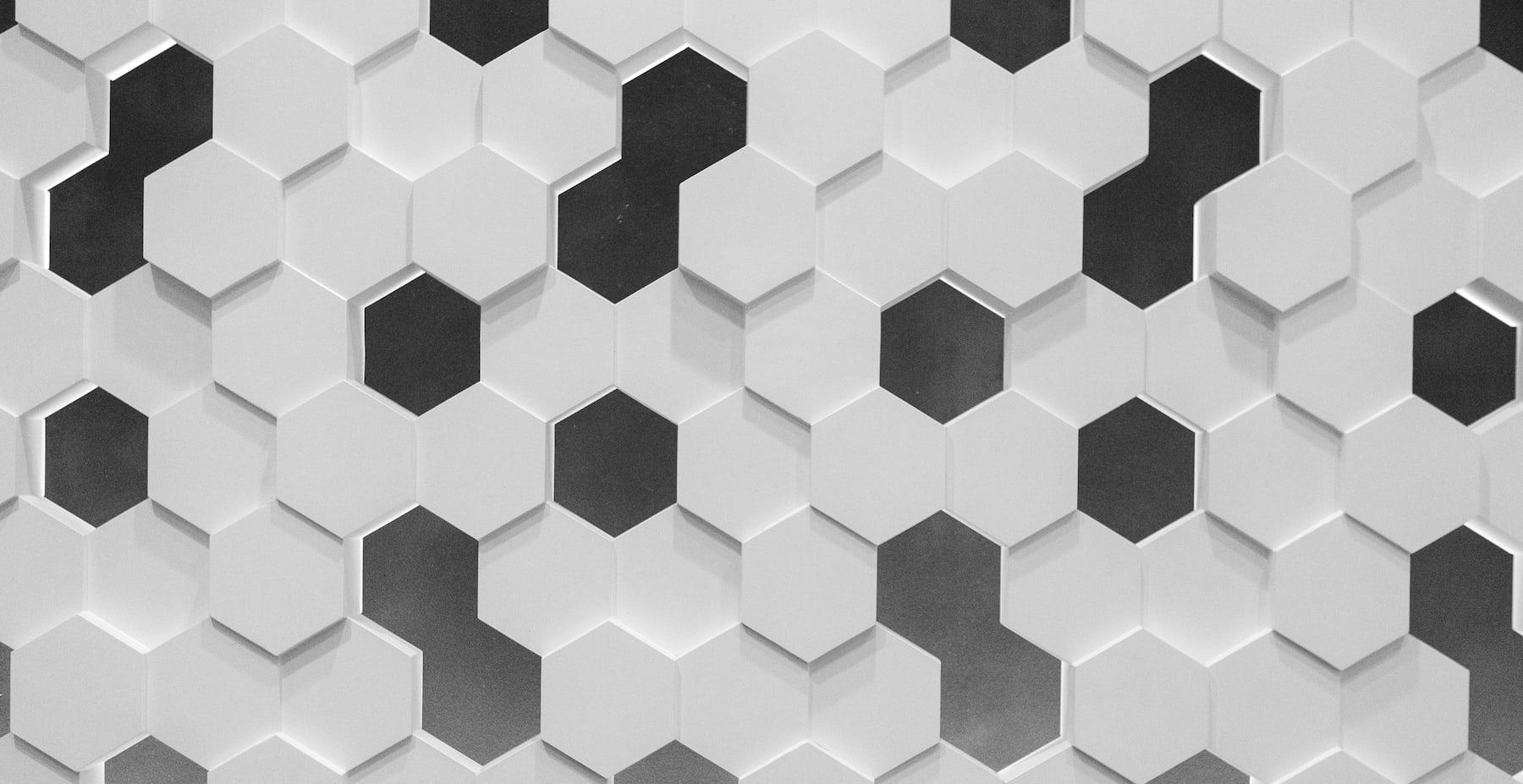If you’re a designer or frontend developer, chances are you’ve happened upon hex color codes (such as `#ff6d91`). Have you ever wondered what the hex you’re looking at when working with hex color codes? In this post we’re going to break down these hex color codes and how they relate to RGB colors.

## What is “hex”?

In our day-to-day life, we generally think and work in a base-10 number system, where the `n`th digit in a number represents some scaled amount of `10^n`. For example, `257` can be thought of as `2*100 + 5*10 + 7*1`. Each time you move a digit to the left, your multiplier is getting 10 times larger. And for each digit place, we need 10 options (0 through 9). This is illustrated below.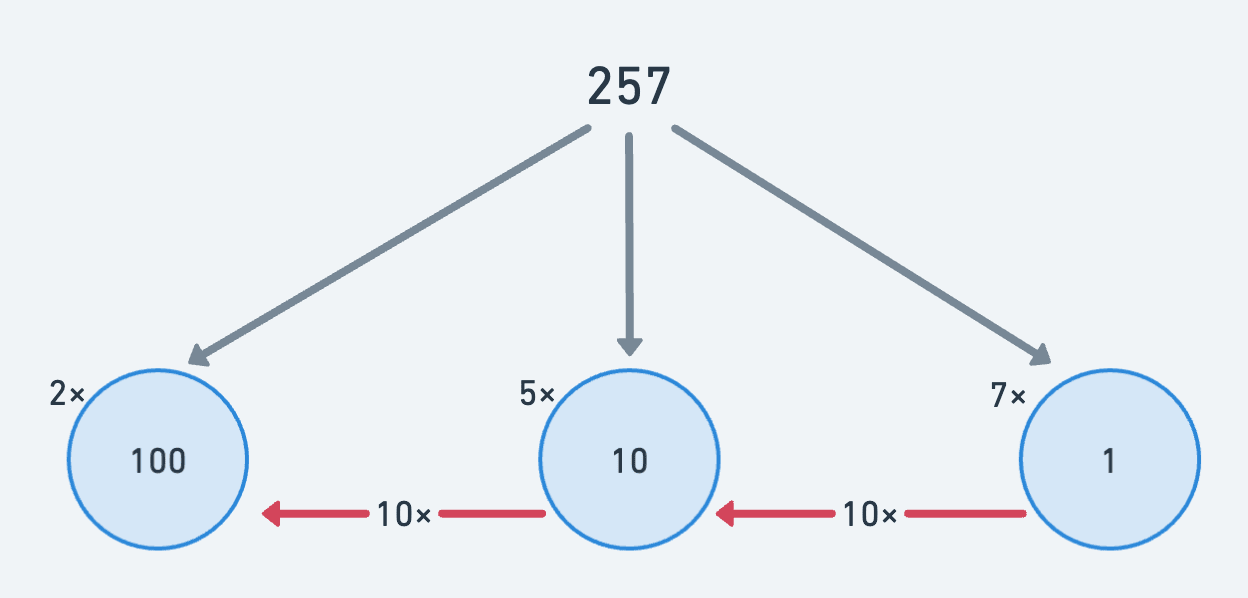When it comes to computing, you might be familiar with the notion of “binary” – which is a base-2 number system. For example, the binary number `0b10110` (where the `0b` prefix just indicates that it’s a binary number) is equal to `1*16 + 0*8 + 1*4 + 1*2 + 0*1 = 22` – notice how each time you move a digit to the left, the “multiplier” becomes 2 times larger, and there are only two options per digit place (`0` and `1`). Therefore `0b10110` is the binary (or base-2) representation of the base-10 number `22`.

Okay, so how do hexadecimals fit into this? Well, hexadecimal numbers are just base-16 numbers. Each digit place in a hexadecimal number needs 16 options… but we only have 0 through 9 for number options. To deal with this issue, we just start dipping into the alphabet and borrow letters “a” through “f”!

Our base-16 digits now run “from 0 to f”, which feels a bit weird to say – but just keep in mind that we’re tacking “a” through “f” onto the end of 0…9.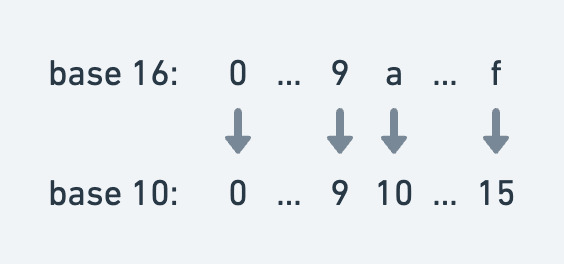Let’s take a peak at a sample hexadecimal number. The number `0x38f` (where the `0x` prefix just indicates that this is a hexadecimal number) is actually equal to `3*16^2 + 8*16 + 15*1 = 911` . Remember, `f` in hex-world is equal to `15` in base-10-world!

Okay, chances are you’re not going to want to do these computations by hand. Let’s use a little JavaScript to move back and forth between hex-world and base-10-world.

### Converting between base-10 and hexadecimal in JS

Fear not, converting between base-10 and hexadecimal in JS is quite easy! There are already built-in functions to handle this for you.

``````const base10ToHex = (x: number) => x.toString(16);
const hexToBase10 = (h: string) => parseInt(h, 16);``````

Yep, that’s it. The `Number.prototype.toString` method accepts a base (or a “radix”) as an argument and will convert the given number to that base. The `parseInt` global function accepts a base (or “radix”) as a second argument and will assume the first argument is a number in that specified base.

Remember our `0x38f` math we did above? I much prefer to open a JS REPL and run `parseInt("38f", 16)`. It returns `911` and I don’t have to do any arithmetic. (You can also just enter `0x38f` into a JS REPL and it’ll give you the base-10 equivalent, but that’s a bit less generalizable.)

Okay, so this is feeling a lot like math. Let’s talk about colors instead.

## Hex color codes and RGB

I’m assuming you have some familiarity with RGB colors, where you can represent a color as `rgb(R, G, B)` where `R,G,B` are integer values between 0 and 255 – and each represent how much of the respective red/green/blue color should be “added” to the resulting color.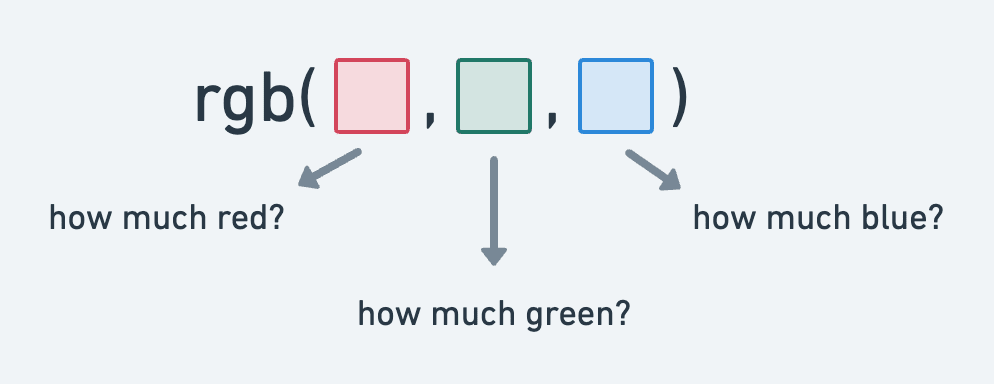Each of the red, green, and blue components can be an integer between 0 and 255 – a total of 256 total options. Conveniently, `256 = 16^2` and therefore you can nicely represent “an integer between 0 and 255” as a 2-digit hexadecimal value! It turns out, that’s pretty much what hex color codes are all about – turning your RGB color representation (using base-10 RGB values) into a similar RGB representation using hexadecimal values! This is best illustrated with a diagram.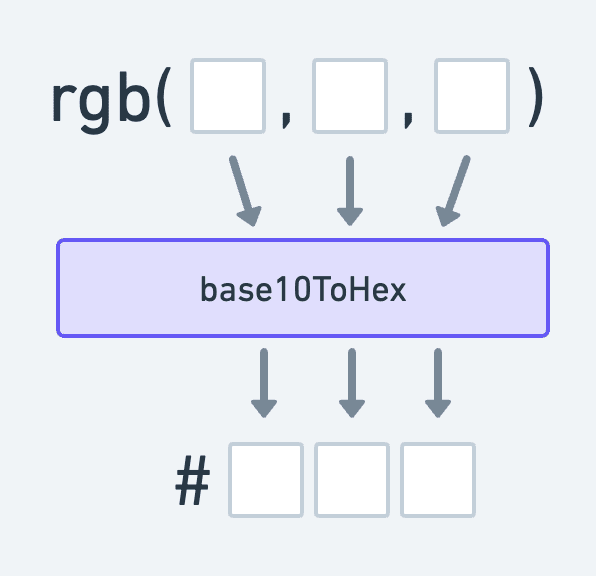For example, `rgb(100, 30, 200)` can be represented as `#641ec8` because when we convert our RGB components from base-10 to hexadecimal, we have `100 → 64` and `30 → 1e` and `200 → c8`. Make sure you can match those up in the two different representations of the color!

To really drive this point home, let’s write a little function that will do this conversion for us.

``````// Note the padStart(2, '0') to ensure length of 2
const base10ToHex = (x: number) => x.toString(16).padStart(2, '0');

const rgbToHex = ({ r, g, b }: { r: number; g: number; b: number }) => {
return `#\${base10ToHex(r)}\${base10ToHex(g)}\${base10ToHex(b)}`;
}``````

I’ve omitted additional checks (to ensure `r`, `g`, and `b` are integers between 0 and 255), but hopefully you get the idea. It’s worth pointing out the added `.padStart(2, '0')` which will ensure that each hex value will have two digits (padding with `0` if necessary).

Since the RGB and hexadecimal representations of colors are in a sense “isomorphic”, we can just as easily move from hex to RGB!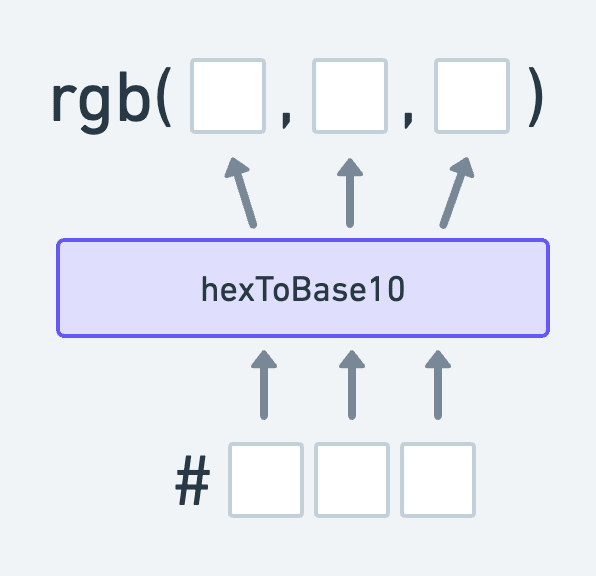Let’s write a little code to do this conversion.

``````// For simplicity, assume hexValue of shape #xxxxxx
const hexToRgb = (hexValue: string) => {
const rHex = hexValue.substring(1, 3);
const gHex = hexValue.substring(3, 5);
const bHex = hexValue.substring(5, 7);

const r = hexToBase10(rHex);
const g = hexToBase10(gHex);
const b = hexToBase10(bHex);

return { r, g, b };
}``````

Awesome! Now we’ve seen enough code to successfully move back and forth between RGB and hex representations.

## 3-digit hex codes

You’ll generally see hex color codes as 6-digit hex codes, such as `#ff6d91`. However, you might also run into 3-digit hex color codes out in the wild!

Recall that 6-digit hex color are just hexadecimal representations of RGB colors, where the first two hex digits (say `r1` and `r2`) represent “how much red”, the second two hex digits (say `g1` and `g2`) represent “how much green”, and the last two hex digits (say `b1` and `b2`) represent “how much blue”.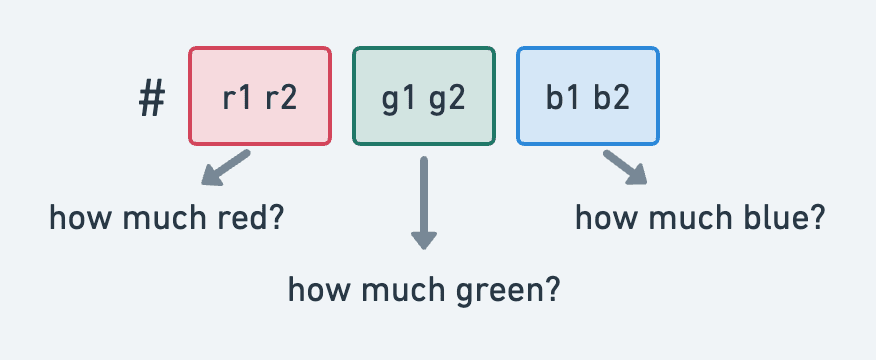There’s a special scenario where `r1 === r2` and `g1 === g2` and `b1 === b2` (such as for `#ff0033`). In this scenario, we can cheekily condense our representation from `#[r1r2][g1g2][b1b2]` to just `#[r1][g1][b1]` – and instead of writing the duplicate digits for each color dimension, just use a single digit!

Here’s an example. Since `#ff0033` has duplicate digits in all three RGB dimensions (e.g. we have `ff` for red, `00` for green, and `33` for blue), we can “fold” the dimensions down into single digits, representing this color as just `#f03`. This is represented below in the diagram below.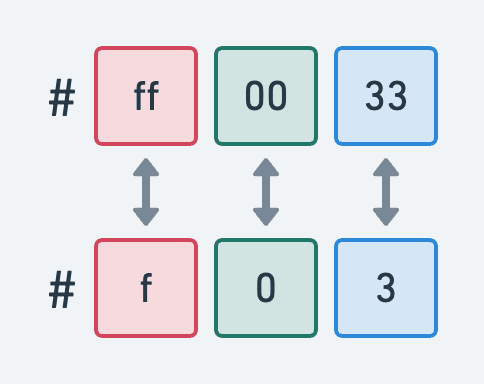In this special scenario, we can “fold” our 6-digit representation down into a 3-digit representation. This also means that if you see a 3-digit hex color code, it’s just a folded-down version of a 6-digit color code and you can expand it back out by duplicating each digit! For example, `#a3f` is really just `#aa33ff` in disguise! Here’s a little code to showcase this expansion.

``````const expandHexColor = (hexCode: string): string => {
const hexValue = hexCode.substring(1);

// If 3-digits, duplicate each digit.
if (hexValue.length === 3) {
const expandedHexValue = [...hexValue].map(x => `\${x}\${x}`).join('')
return `#\${expandedHexValue}`;
}

// Otherwise, we'll assume it's a 6-digit code and return the original.
return hexCode;
}``````

## Alpha channel: 8-digit hex codes

Browsers support a “fourth dimension” for RGB colors, namely the “alpha channel” – which specifies how transparent/opaque the color is (or, how “see-through” it is). In CSS, you’ll generally see this specified via the `rgba` function (the trailing `a` standing for “alpha”), where you can specify how much opacity the color should have (where `a` varies from 0 to 1).

For example, `rgb(21, 188, 168)` is a blueish color. If we wanted to make this “partially opaque”, we could specify an opacity of 0.6 by writing `rgba(21, 188, 168, 0.6)`.

Hex color codes can also support alpha channel specification! If you take a 6-digit hex color code, such as `#ff0033`, you can specify the opacity by adding two more hex digits to the end to specify the alpha channel amount – where `00` (`0` in base-10) is no opacity and `ff` (`255` in base-10) is full opacity. As an example `#ff003380` is just `#ff0033` with partial opacity.

With `rgba`, the alpha dimension ranges from 0 to 1. However, with 8-digit hex color codes, the alpha dimension ranges from 0 to 255 – and therefore when converting back and forth between `rgba` and 8-digit hex color codes, you’ll have to scale up or down by 255 accordingly.

We can now update our hex ↔ RGB conversions to handle alpha channel!

``````const rgbaToHex = ({ r, g, b, a = 1 }: { r: number; g: number; b: number; a?: number }) => {
const hex = `#\${base10ToHex(r)}\${base10ToHex(g)}\${base10ToHex(b)}`;

// If a (opacity) is 1, use 6-digit hex code
if (a === 1) return hex;

// Otherwise, scale a by 255 and convert to hex for the alpha-channel digits.
return `\${hex}\${base10ToHex(Math.round(255 * a))}`
}

const hexToRgba = (hexValue: string) => {
const rHex = hexValue.substring(1, 3);
const gHex = hexValue.substring(3, 5);
const bHex = hexValue.substring(5, 7);
// alpha-channel, default to 'ff' (full opacity)
const aHex = hexValue.substring(7, 9) || 'ff';

const r = hexToBase10(rHex);
const g = hexToBase10(gHex);
const b = hexToBase10(bHex);
// Remember to scale from [0, 255] to [0, 1]
const a = hexToBase10(aHex) / 255;

return { r, g, b, a };
}``````

Notice how we have to scale the alpha channel up/down by 255 and convert between base-10 and hex.

### 4-digit hex codes

Just like how we can, by convention, “fold” some special 6-digit hex color codes into 3-digit ones, we can do a similar thing with 8-digit hex color codes! We can do this whenever the RGB components of the hex code (the first 6 digits) are “foldable” and the last 2 digits (that specify the alpha channel amount) are also “foldable”.

As an example, `#ff003388` can be “folded” all the way down to `#f038`. However, `#ff003380` cannot be folded to a 4-digit representation because the alpha-channel digits `80` are not the same.

## Wrap up

So many options! When using hex color codes, we can have 3-digit, 4-digit, 6-digit, or 8-digit hex color codes! This is a lot of edge cases to handle if you’re writing conversion code, but luckily most design and development tools handle this sort of thing for you. If in doubt, just stick to 6-digit hex codes – or use 8-digit hex codes if you need to specify an opacity.

Color theory is complex. This post will not make you a color expert. However, if you’re a designer or frontend developer, chances are you’re working with hex color codes regularly. Having a baseline understanding of what this color representation actually means might help you in understanding the hex color codes you’re using on a daily basis!

## Related Posts

### Scaling with Confidence: Next Generation Query Building with GROQD and Sanity

July 14, 2023
Introducing GROQD: zero build step query composition, runtime validation & type-safety all declared in one place.

### Mock factories make better tests

February 20, 2023
Developers need a good way to produce mock data. Here’s how we implemented a mock factory for an e-commerce site that’s simple, flexible, and fun.